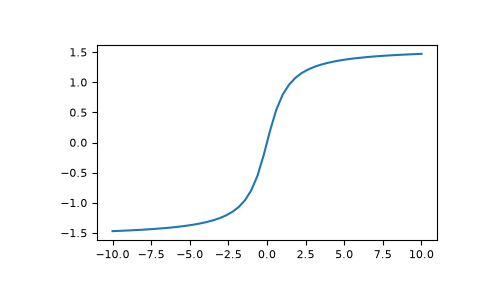# numpy.arctan¶

`numpy.``arctan`(x, /, out=None, *, where=True, casting='same_kind', order='K', dtype=None, subok=True[, signature, extobj]) = <ufunc 'arctan'>

Trigonometric inverse tangent, element-wise.

The inverse of tan, so that if `y = tan(x)` then `x = arctan(y)`.

Parameters
xarray_like
outndarray, None, or tuple of ndarray and None, optional

A location into which the result is stored. If provided, it must have a shape that the inputs broadcast to. If not provided or None, a freshly-allocated array is returned. A tuple (possible only as a keyword argument) must have length equal to the number of outputs.

wherearray_like, optional

This condition is broadcast over the input. At locations where the condition is True, the out array will be set to the ufunc result. Elsewhere, the out array will retain its original value. Note that if an uninitialized out array is created via the default `out=None`, locations within it where the condition is False will remain uninitialized.

**kwargs

For other keyword-only arguments, see the ufunc docs.

Returns
outndarray or scalar

Out has the same shape as x. Its real part is in `[-pi/2, pi/2]` (`arctan(+/-inf)` returns `+/-pi/2`). This is a scalar if x is a scalar.

`arctan2`

The “four quadrant” arctan of the angle formed by (x, y) and the positive x-axis.

`angle`

Argument of complex values.

Notes

`arctan` is a multi-valued function: for each x there are infinitely many numbers z such that tan(z) = x. The convention is to return the angle z whose real part lies in [-pi/2, pi/2].

For real-valued input data types, `arctan` always returns real output. For each value that cannot be expressed as a real number or infinity, it yields `nan` and sets the invalid floating point error flag.

For complex-valued input, `arctan` is a complex analytic function that has [1j, infj] and [-1j, -infj] as branch cuts, and is continuous from the left on the former and from the right on the latter.

The inverse tangent is also known as atan or tan^{-1}.

References

Abramowitz, M. and Stegun, I. A., Handbook of Mathematical Functions, 10th printing, New York: Dover, 1964, pp. 79. http://www.math.sfu.ca/~cbm/aands/

Examples

We expect the arctan of 0 to be 0, and of 1 to be pi/4:

```>>> np.arctan([0, 1])
array([ 0.        ,  0.78539816])
```
```>>> np.pi/4
0.78539816339744828
```

Plot arctan:

```>>> import matplotlib.pyplot as plt
>>> x = np.linspace(-10, 10)
>>> plt.plot(x, np.arctan(x))
>>> plt.axis('tight')
>>> plt.show()
```